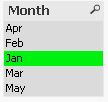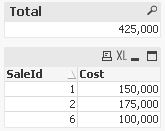QlikView App Dev

Discussion Board for collaboration related to QlikView App Development.

Announcements
QlikWorld 2022, LIVE in Denver CO., May 16-19, 2022. REGISTER NOW TO RECEIVE EARLY BIRD PRICING
cancel
Showing results for
Did you mean:Not applicable

How to update the value based on a range in the another table or variable?

Given two files,where sales data contains the details of the sales according to ID or month.

Commission data gives the commission percentages according to ranges.

Now my problem is,When I select a month field in the sheet,if they are more than one sales in that month,two fields get select in the commission percentage if they belong to different ranges.

What I need is instead of that,depending the select values in the sales field,it should calculate the total costs of the sales and check the range and highlight the commission matrix the corresponding percentage.

1 Solution

Accepted SolutionsMVP

Hi,

one solution without creating a static interval matching table could be to use calculated listboxes with expressions like:

=Aggr(Only({\$<Commision={'=[Low Value]<=Sum(Cost) and [High Value]>=Sum(Cost)'}>} Commision), Commision)

or

=Aggr(If(Sum(Cost)>=[Low Value] and Sum(Cost)<=[High Value],Commision),Commision)hope this helps

regards

Marco

3 RepliesMVP

Hi,

one solution without creating a static interval matching table could be to use calculated listboxes with expressions like:

=Aggr(Only({\$<Commision={'=[Low Value]<=Sum(Cost) and [High Value]>=Sum(Cost)'}>} Commision), Commision)

or

=Aggr(If(Sum(Cost)>=[Low Value] and Sum(Cost)<=[High Value],Commision),Commision)hope this helps

regards

MarcoNot applicable
Author

Hi Marco,

Thanks a lot.This is exactly what I was looking for.

Thanks and regards,

SrinathMVP